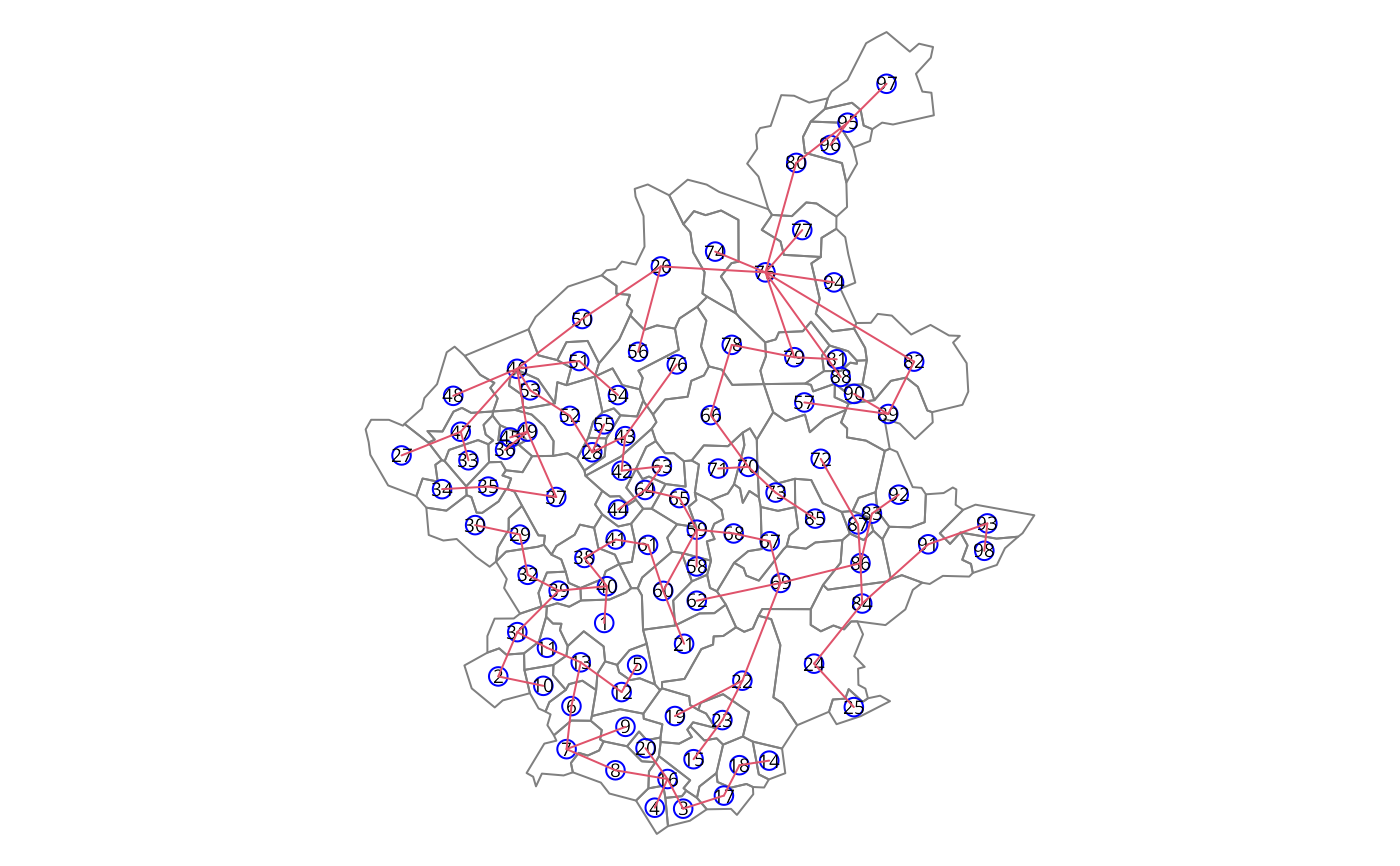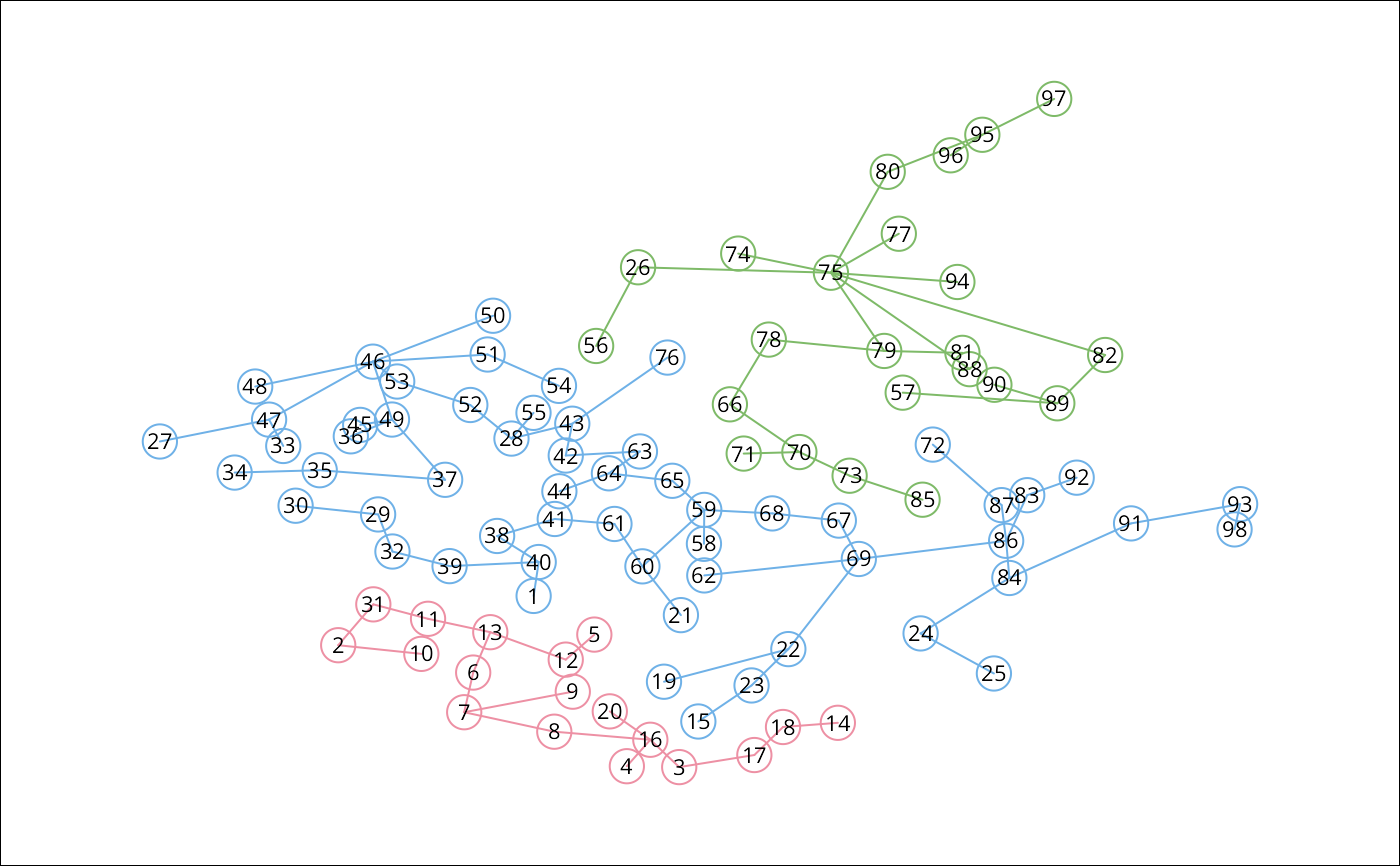This function implements a SKATER procedure for spatial clustering analysis. This procedure essentialy begins with an edges set, a data set and a number of cuts. The output is an object of 'skater' class and is valid for input again.

skater(edges, data, ncuts, crit, vec.crit, method = c("euclidean",
"maximum", "manhattan", "canberra", "binary", "minkowski",
"mahalanobis"), p = 2, cov, inverted = FALSE)

## Arguments

edges

A matrix with 2 colums with each row is an edge

data

A data.frame with data observed over nodes.

ncuts

The number of cuts

crit

A scalar or two dimensional vector with criteria for groups. Examples: limits of group size or limits of population size. If scalar, is the minimum criteria for groups.

vec.crit

A vector for evaluating criteria.

method

Character or function to declare distance method. If method is character, method must be "mahalanobis" or "euclidean", "maximum", "manhattan", "canberra", "binary" or "minkowisk". If method is one of "euclidean", "maximum", "manhattan", "canberra", "binary" or "minkowski", see dist for details, because this function as used to compute the distance. If method="mahalanobis", the mahalanobis distance is computed between neighbour areas. If method is a function, this function is used to compute the distance.

p

The power of the Minkowski distance.

cov

The covariance matrix used to compute the mahalanobis distance.

inverted

logical. If 'TRUE', 'cov' is supposed to contain the inverse of the covariance matrix.

## Value

A object of skater class with:

groups

A vector with length equal the number of nodes. Each position identifies the group of node

edges.groups

A list of length equal the number of groups with each element is a set of edges

not.prune

A vector identifying the groups with are not candidates to partition.

candidates

A vector identifying the groups with are candidates to partition.

ssto

The total dissimilarity in each step of edge removal.

## Author

Renato M. Assuncao and Elias T. Krainski

See Also as mstree

## Examples

### loading data
package="spdep"), quiet=TRUE)
st_crs(bh) <- "OGC:CRS84"
### data standardized

### neighboorhod list
bh.nb <- poly2nb(bh)

### calculating costs

### making listw
nb.w <- nb2listw(bh.nb, lcosts, style="B")

### find a minimum spanning tree
mst.bh <- mstree(nb.w,5)

### the mstree plot
par(mar=c(0,0,0,0))
plot(st_geometry(bh), border=gray(.5))
pts <- st_coordinates(st_centroid(bh))
#> Warning: st_centroid assumes attributes are constant over geometries
plot(mst.bh, pts, col=2,### three groups with no restriction
#> Error in if (w$num_obs < 1) { stop("The weights is not valid.")}: argument is of length zero ### groups size table(res1$groups)

### the skater plot
opar <- par(mar=c(0,0,0,0))
plot(res1, pts, cex.circles=0.035, cex.lab=.7)
#> Error in h(simpleError(msg, call)): error in evaluating the argument 'x' in selecting a method for function 'plot': object 'res1' not found

### the skater plot, using other colors
plot(res1, pts, cex.circles=0.035, cex.lab=.7,
groups.colors=heat.colors(length(res1$ed))) #> Error in h(simpleError(msg, call)): error in evaluating the argument 'x' in selecting a method for function 'plot': object 'res1' not found ### the Spatial Polygons plot plot(st_geometry(bh), col=heat.colors(length(res1$edg))[res1$groups]) #> Error in eval(expr, envir, enclos): object 'res1' not foundpar(opar) ### EXPERT OPTIONS ### more one partition res1b <- skater(res1, dpad, 1) #> Error in if (w$num_obs < 1) {    stop("The weights is not valid.")}: argument is of length zero

### length groups frequency
table(res1$groups) #> Error in eval(expr, envir, enclos): object 'res1' not found table(res1b$groups)

### thee groups with minimum population
res2 <- skater(mst.bh[,1:2], dpad, 2, 200000, bh$Pop) #> Error in if (w$num_obs < 1) {    stop("The weights is not valid.")}: argument is of length zero
table(res2$groups) #> Error in eval(expr, envir, enclos): object 'res2' not found ### thee groups with minimun number of areas res3 <- skater(mst.bh[,1:2], dpad, 2, 3, rep(1,nrow(bh))) #> Error in if (w$num_obs < 1) {    stop("The weights is not valid.")}: argument is of length zero
table(res3$groups) #> Error in eval(expr, envir, enclos): object 'res3' not found ### thee groups with minimun and maximun number of areas res4 <- skater(mst.bh[,1:2], dpad, 2, c(20,50), rep(1,nrow(bh))) #> Error in if (w$num_obs < 1) {    stop("The weights is not valid.")}: argument is of length zero
table(res4$groups) #> Error in eval(expr, envir, enclos): object 'res4' not found ### if I want to get groups with 20 to 40 elements res5 <- skater(mst.bh[,1:2], dpad, 2, c(20,40), rep(1,nrow(bh))) ## DON'T MAKE DIVISIONS #> Error in if (w$num_obs < 1) {    stop("The weights is not valid.")}: argument is of length zero
table(res5$groups) #> Error in eval(expr, envir, enclos): object 'res5' not found ### In this MST don't have groups with this restrictions ### In this case, first I do one division ### with the minimun criteria res5a <- skater(mst.bh[,1:2], dpad, 1, 20, rep(1,nrow(bh))) #> Error in if (w$num_obs < 1) {    stop("The weights is not valid.")}: argument is of length zero
table(res5a$groups) #> Error in eval(expr, envir, enclos): object 'res5a' not found ### and do more one division with the full criteria res5b <- skater(res5a, dpad, 1, c(20, 40), rep(1,nrow(bh))) #> Error in if (w$num_obs < 1) {    stop("The weights is not valid.")}: argument is of length zero
table(res5b$groups) #> Error in eval(expr, envir, enclos): object 'res5b' not found ### and do more one division with the full criteria res5c <- skater(res5b, dpad, 1, c(20, 40), rep(1,nrow(bh))) #> Error in if (w$num_obs < 1) {    stop("The weights is not valid.")}: argument is of length zero
table(res5c$groups) #> Error in eval(expr, envir, enclos): object 'res5c' not found ### It don't have another divison with this criteria res5d <- skater(res5c, dpad, 1, c(20, 40), rep(1,nrow(bh))) #> Error in if (w$num_obs < 1) {    stop("The weights is not valid.")}: argument is of length zero
table(res5d$groups) #> Error in eval(expr, envir, enclos): object 'res5d' not found # \dontrun{ data(boston, package="spData") bh.nb <- boston.soi dpad <- data.frame(scale(boston.c[,c(7:10)])) ### calculating costs system.time(lcosts <- nbcosts(bh.nb, dpad)) #> user system elapsed #> 0.039 0.000 0.040 ### making listw nb.w <- nb2listw(bh.nb, lcosts, style="B") ### find a minimum spanning tree mst.bh <- mstree(nb.w,5) ### three groups with no restriction system.time(res1 <- skater(mst.bh[,1:2], dpad, 2)) #> Error in if (w$num_obs < 1) {    stop("The weights is not valid.")}: argument is of length zero
#> Timing stopped at: 0 0 0
library(parallel)
nc <- max(2L, detectCores(logical=FALSE), na.rm = TRUE)-1L
# set nc to 1L here
if (nc > 1L) nc <- 1L
coresOpt <- get.coresOption()
invisible(set.coresOption(nc))
if(!get.mcOption()) {
# no-op, "snow" parallel calculation not available
cl <- makeCluster(get.coresOption())
set.ClusterOption(cl)
}
### calculating costs
#>    user  system elapsed
#>   0.038   0.000   0.038
all.equal(lcosts, plcosts, check.attributes=FALSE)
#>  TRUE
### making listw
pnb.w <- nb2listw(bh.nb, plcosts, style="B")
### find a minimum spanning tree
pmst.bh <- mstree(pnb.w,5)
### three groups with no restriction